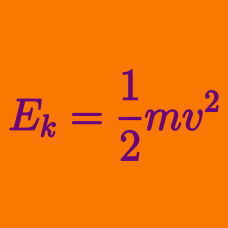Classical Mechanics

# Kinetic energy of an object

A $14\text{ kg}$ object initially at rest starts to fall freely. If air resistance is negligible, what is the object's kinetic energy after $0.5$ seconds?

The gravitational acceleration is $g=10\text{ m/s}^2.$

What is the kinetic energy of a $6\text{ kg}$ object moving at $10\text{ m/s}?$

A car of mass $1900\text{ kg}$ is traveling at $28\text{ m/s}$ on a street that has a speed limit of $20\text{ m/s}.$ The driver spots a police car and reduces its speed to match the speed limit. How much kinetic energy did the car lose?

A $10\text{ kg}$ object, $A$, travels at $6\text{ m/s},$ and a $6\text{ kg}$ object, $B$, travels at $10\text{ m/s}.$

Find the ratio $\text{K.E.}_A/\text{K.E.}_B$.

Recently, a meteor impacted Russia. The estimate of the meteor's size was $10~\mbox{tons}$ and it was estimated to have entered the earth's atmosphere at a speed of $14,600~\mbox{m/s}$. What was the total kinetic energy of such a meteor $\si{\joule}$ as it entered the atmosphere?

Details and assumptions

• One ton is $907~\mbox{kg}$.
×